Symbol
ProblemActivity $1:$ Transform each of the following equations into a quadratic equation in the form of $ax^{2}+bx+c=0$ and find the solution set. $1.$ $\times \left(x+3\right)=28$ $2.$ $3s\left(5-2\right)=125$ $3.$ $\left(t+1\right)^{2}+\left(t-8\right)^{2}=45$ $4.$ $\left(3r+1\right)^{2}+\left(r+2\right)^{2}=65$ $5.$ $\dfrac {4} {t-3}+\dfrac {t} {2}=-2$
7th-9th grade
Algebra
Search count: 116
Question content
Sir can u pls help my math problem Thanks
Solution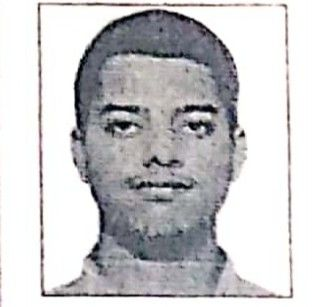Qanda teacher - amir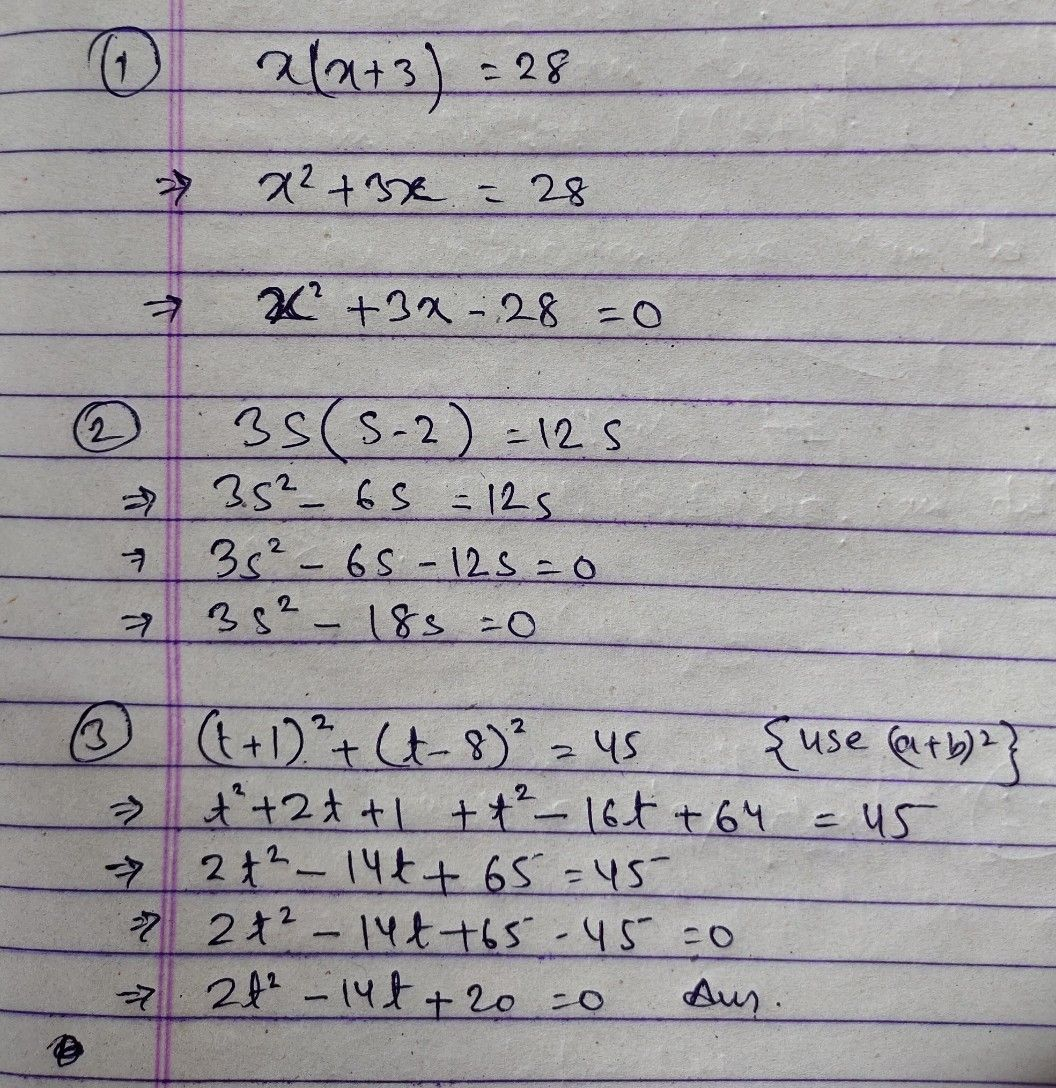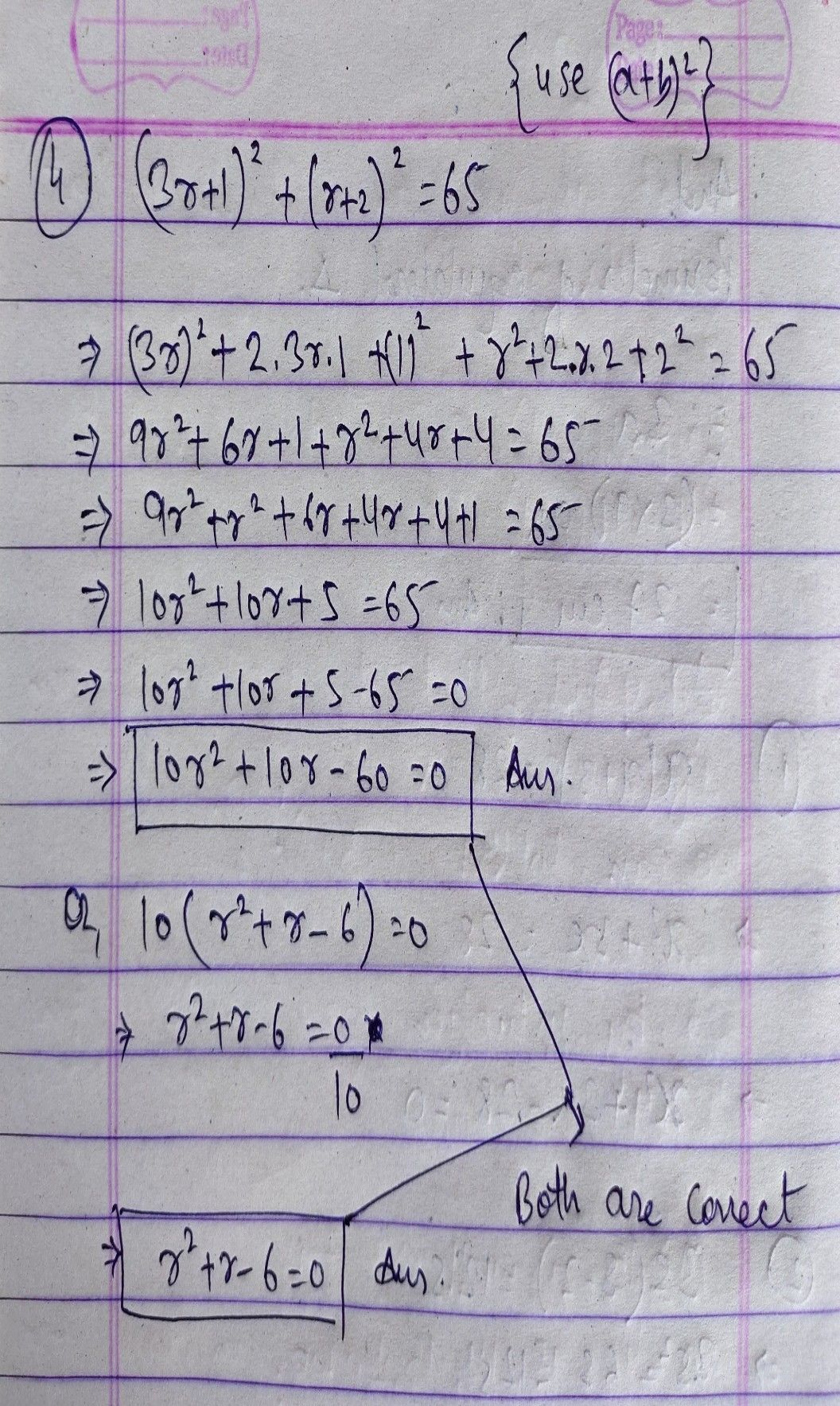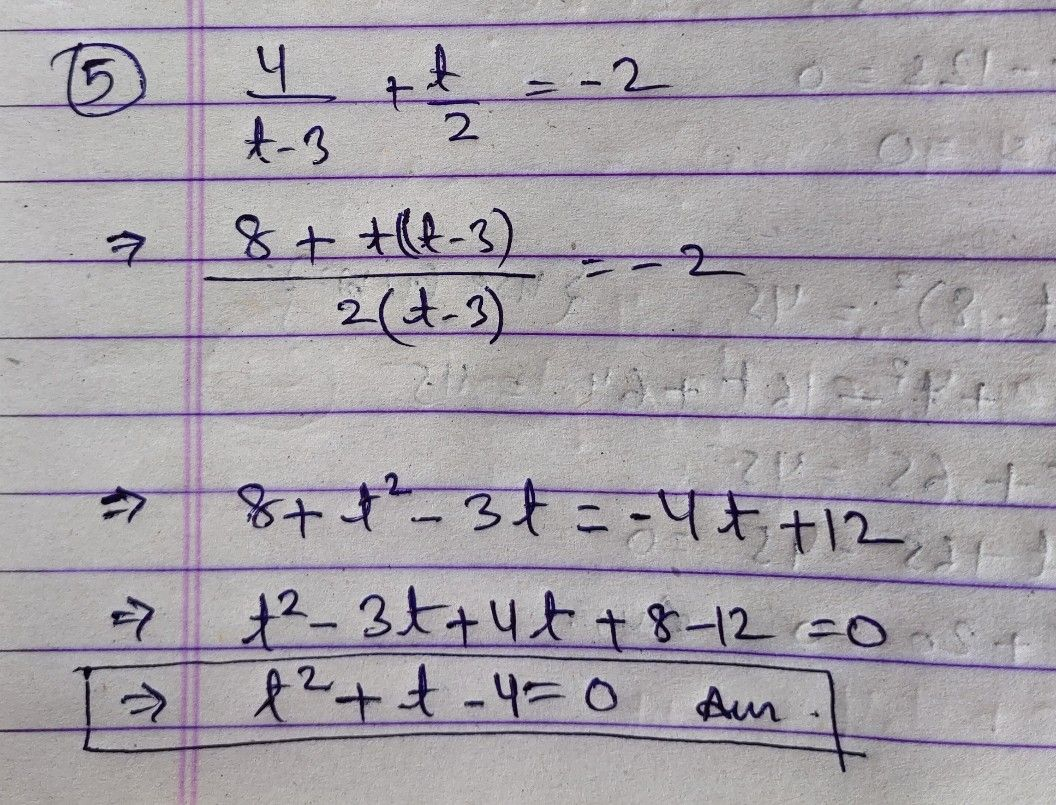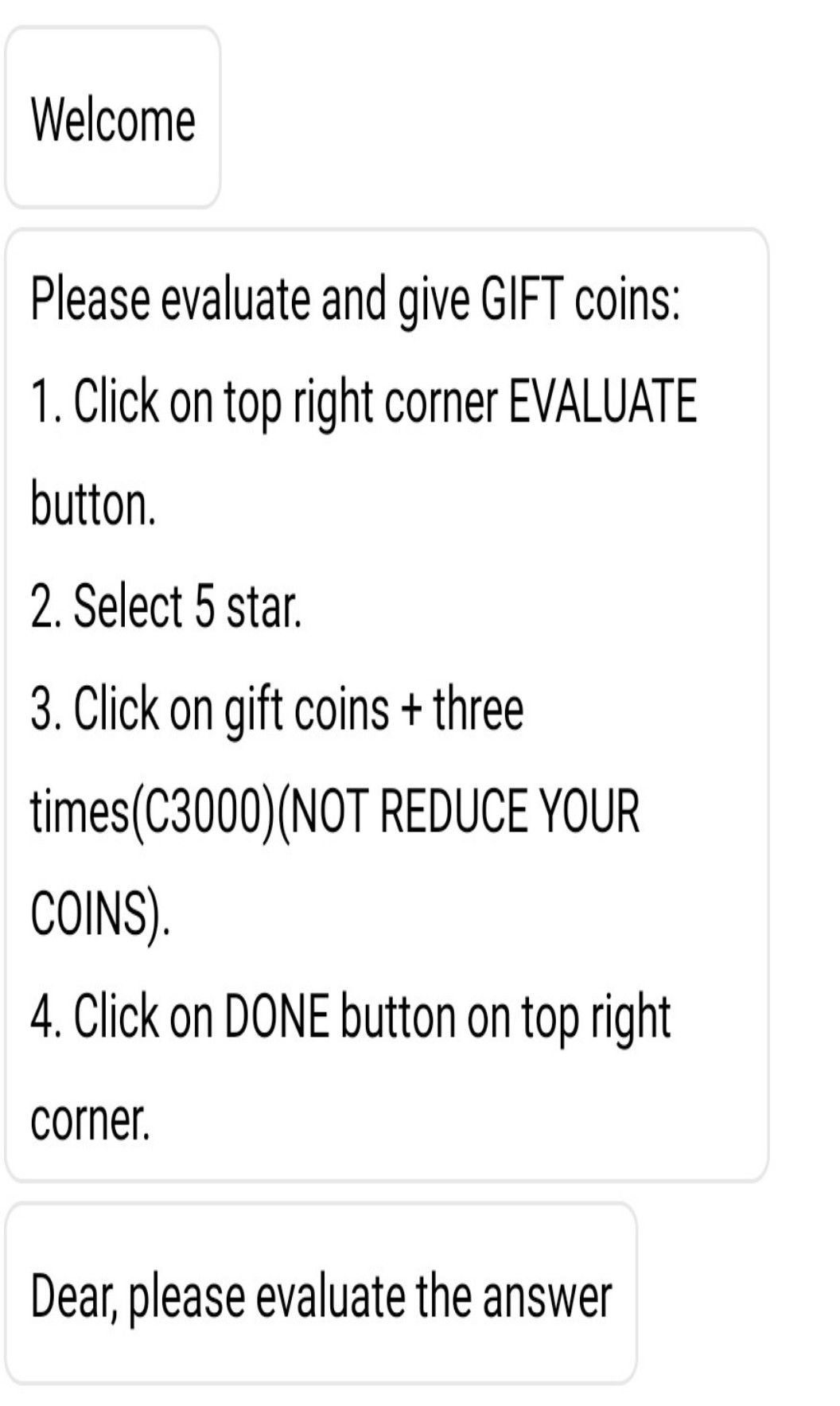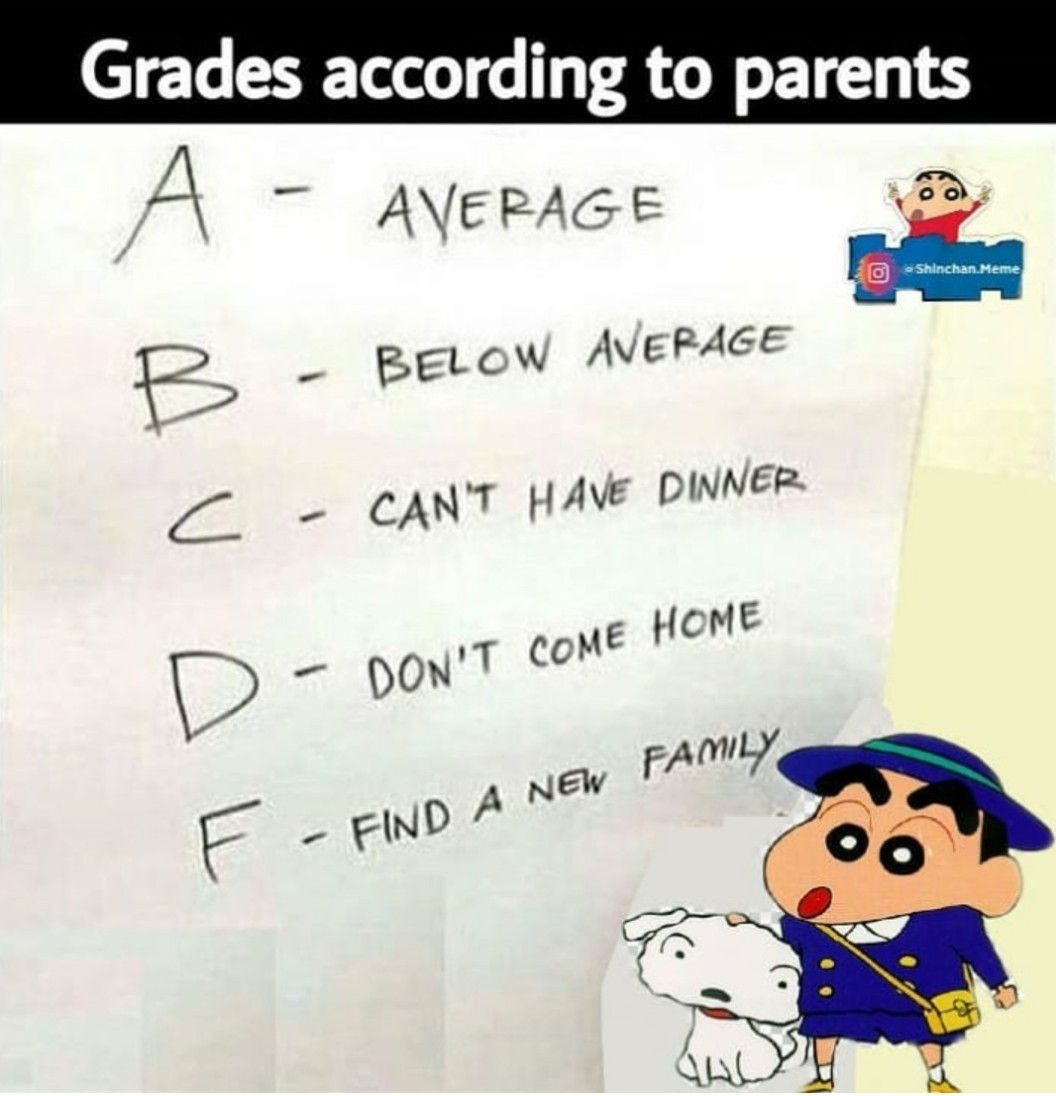Have a nice day dearStudent
Thanks sirQanda teacher - amir
you're welcome dearStudent
Have a good day to you too sir